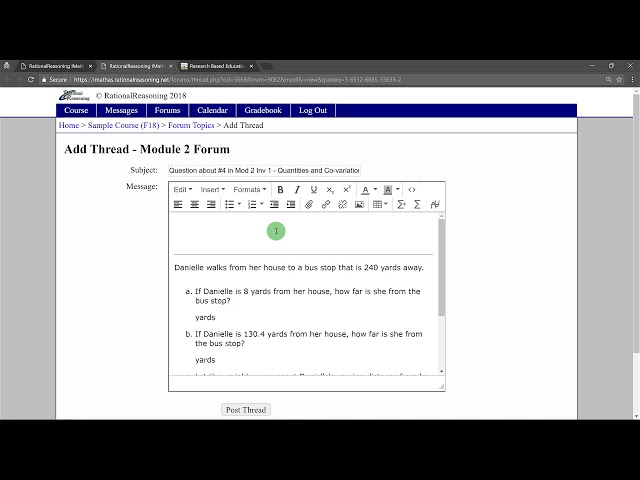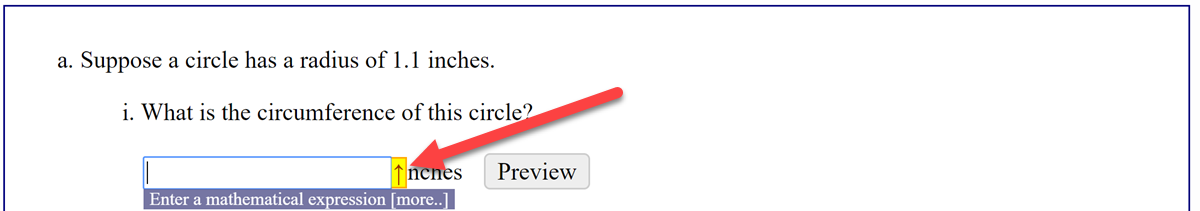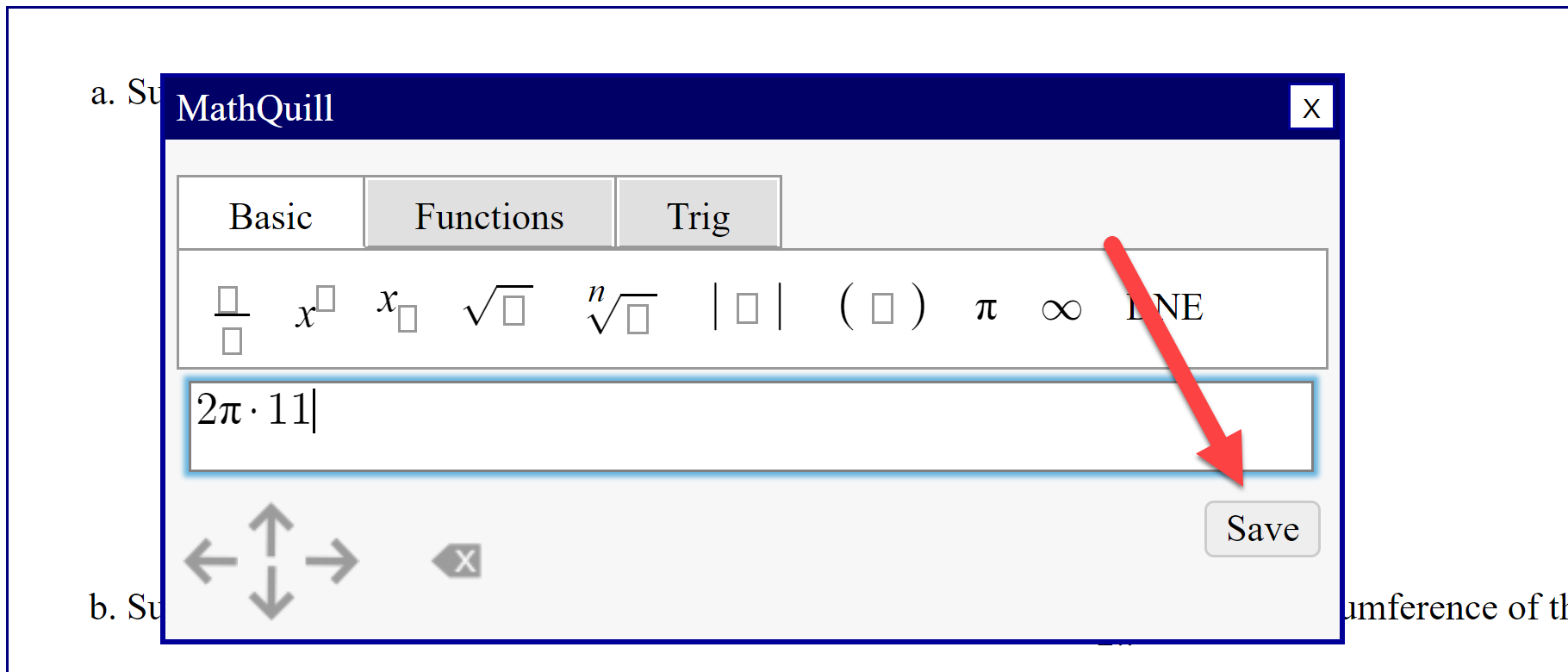# # IMathAS (For Students)

This page provides some help for students new to using the IMathAS online homework system.

TIP

As a student you should, at the very least, watch the first video on this page so that you understand the basics of how to use the IMathAS system.

## # Overview

The video below gives you an overview of how to use the IMathAS system.## # Typing Math

If you've never used an online homework system before, entering your mathematical answers into the online system might seem strange at first. However, the way you type math into your graphing calculator is very similar to how you should type it into iMathAS. Here is a table showing how to enter various mathematical expressions/operators.

EntryMeaningExample
+,-,*,/Add, subtract, multiply, divide"5+2*3-10" gives $5+2\cdot 3-10$ and "3/5" gives $\frac{3}{5}$
^Power or exponent"2^3" gives $2^3$
( )Parentheses for grouping"1/2*pi" gives $\frac{1}{2}\cdot\pi$ while "1/(2*pi)" gives $\frac{1}{2\pi}$
e, piStandard constants $e$ and $\pi$"2 pi r" gives $2\pi r$ and "e^3" gives $e^3$
x, y, t, etc.Variable names"3*x^2" gives $3\cdot x^2$
_Subscript, for variable and function names"t_1" gives $t_1$ and "log_b(y)" gives $\log_b(y)$
absAbsolute Value"abs(-4)" gives $\lvert-4\rvert$
sin, cos, tan, sec, csc, cot, sinh, coshStandard trig. functions"sin(2)" gives $\sin(2)$
arcsin, arccos, arctanInverse trig. functions"arccos(0.5)" gives $\arccos(0.5)$
sin^-1, cos^-1, tan^-1Inverse trig. functions"sin^-1(0.5)" gives $\sin^{-1}(0.5)$
log_bLog with base $b$"log_2(4)" gives $\log_2(4)$
ln, logNatural (base $e$) and common (base 10) log"ln(3)" gives $\ln(3)$
!Factorial"4! = 24" gives $4! = 24$
ooInfinity. Two lower case O's like the middle of the word "book""(-oo, oo)" gives $(-\infty, \infty)$

### # Visual Math Editor

IMathAS also provides a visual interface for entering mathematical answers. If your cursor is in an input box, you should generally see a yellow rectangle with an arrow in it, as shown below.If you click on that arrow, it will pull open a visual math editor. You can click on the tools in the toolbar to help you enter your math. When you're finished with the visual editor, press the "Save" button to save your input.## # Why is that Wrong?

By default, for numerical questions, IMathAS checks to see if your answer is within 0.1% of the exact answer - if it is, you're answer is considered "correct." THIS IS VERY IMPORTANT.

WARNING

Suppose the answer to a question is $\frac{1}{3}$. If you enter this into your calculator you might be tempted to round to 0.33. But 0.33 is actually 1% away from $\frac{1}{3}$ so your answer will be counted incorrect! Instead, enter 0.333333. Or even better! Enter "1/3" and IMathAS will do the computation for you.